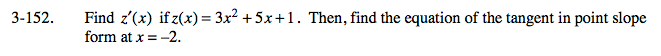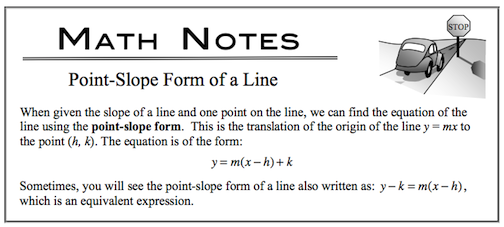### Home > CALC > Chapter Ch3 > Lesson 3.4.1 > Problem3-152

3-152.

Find z′(x) if z(x) = 3x2 + 5x + 1. Then, find the equation of the tangent in point slope form at x = −2. Homework Help ✎To find h and k (the point): h is given as x = −2, therefore k is z(−2).

To find m (the slope):evaluate z'(x) at x = −2.